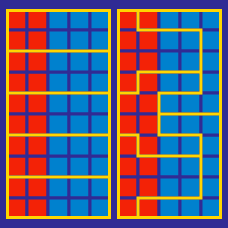Logic

# Gerrymandering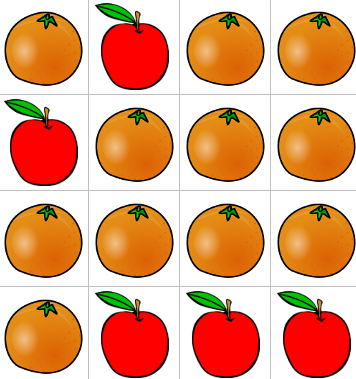The picture above needs to be divided into two parts of equal area by cutting along the grid lines. Here's an example: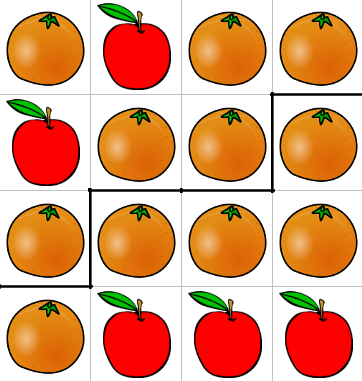Note that in both parts, the oranges outnumber the apples.

Is it possible to divide the picture into two parts of equal area in which the apples outnumber the oranges in one of the parts?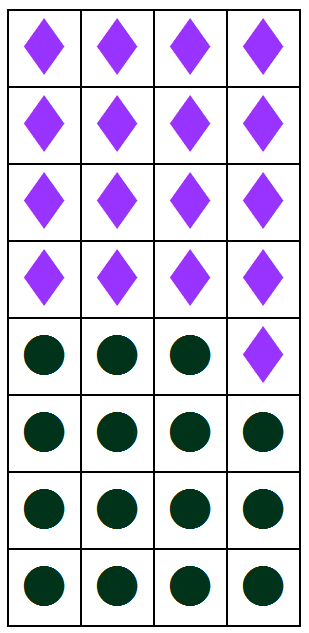The diamonds and dots represent voters. The voters are divided into four districts of equal area, with lines drawn along the grid.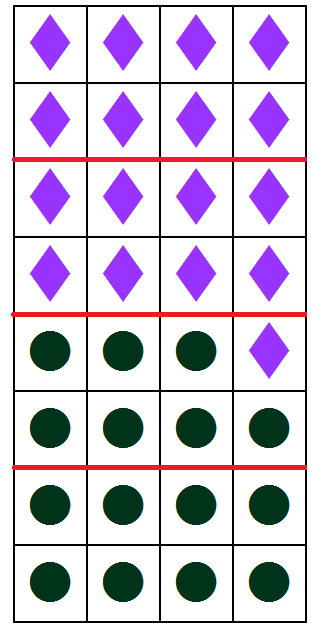In the example above, the dots will win two out of the four districts in a vote.

Suppose the dividing lines were drawn elsewhere. What's the maximum number of districts that the dots can win?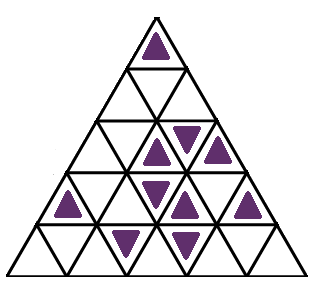Is there a way to divide the grid along the lines into 5 portions of equal area such that there are only two purple triangles in each portion?

NOTE: Every part of the grid will be in one of the 5 portions.

Also, portions of equal area are continuous, and if two areas are connected by only a point they are considered separate.

Proposition 888 is up for vote in the North District, the East District, and the South District. (Ties force the vote to be redone.)

 Total For Against North 50000 20000 30000 East 30000 10000 20000 South 80000 ? ?

If the majority of the votes are For in two of the districts, the measure passes. Clearly the measure is going to fail, but it turns out a majority of voters combined might have voted For anyway!

What's the maximum number of votes the South could have For such that a majority of all the voters combined together still voted Against?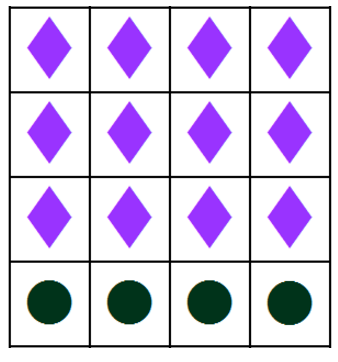The diamonds and dots represent voters. Suppose the voters are divided into four districts of equal area, with lines drawn along the grid. What's the maximum number of districts that the dots can outnumber the diamonds and win?

×